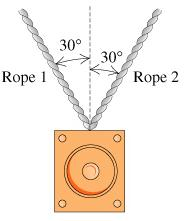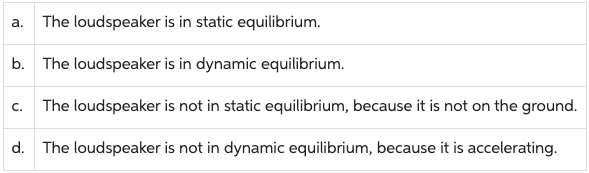# Problem: 1. Which of the following statements are correct about the situation described in the introduction?Check all that apply.2. What forces are acting on this object?

###### FREE Expert Solution

1.

From the given diagram, the loudspeaker is in static equilibrium.

90% (31 ratings)###### Problem Details1. Which of the following statements are correct about the situation described in the introduction?

Check all that apply.2. What forces are acting on this object?